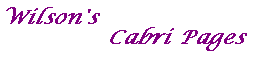#### Proof of Theorem 4

Theorem 4
Suppose that C: xTMx=0 is a non-degenerate conic and that U is any point.
Then the pair of tangents to C from U has equation uTMu xTMx =uTMx uTMx .

Proof
Suppose that the tangent at V=[v] on C passes through U=[u].
Then vTMu=0, and, transposing, uTMv=0.
Also, as V is on C, vTMv=0.
Any point T on the line UV may be written as T=[t], where t=au+bv, for some a, b.
Expanding each side, and using the equalities above,
we see that t satisfies the equation uTMu xTMx =uTMx uTMx .
Similarly, if the tangent at W passes through U, then each point of UW satisfies the equation.
Finally, the given equation is quadratic in x, so can represent no more than two lines.

Of course, by La Hire's Theorem, the chord VW in the above theorem is the polar of U.